CBSE Class 12 Sample Paper for 2019 Boards

Class 12
Solutions of Sample Papers and Past Year Papers - for Class 12 Boards

Question 22

If vector p = i + j + k and q = i – 2j + k, find a vector of magnitude 5√3 units perpendicular to the vector q and coplanar with vectors p and q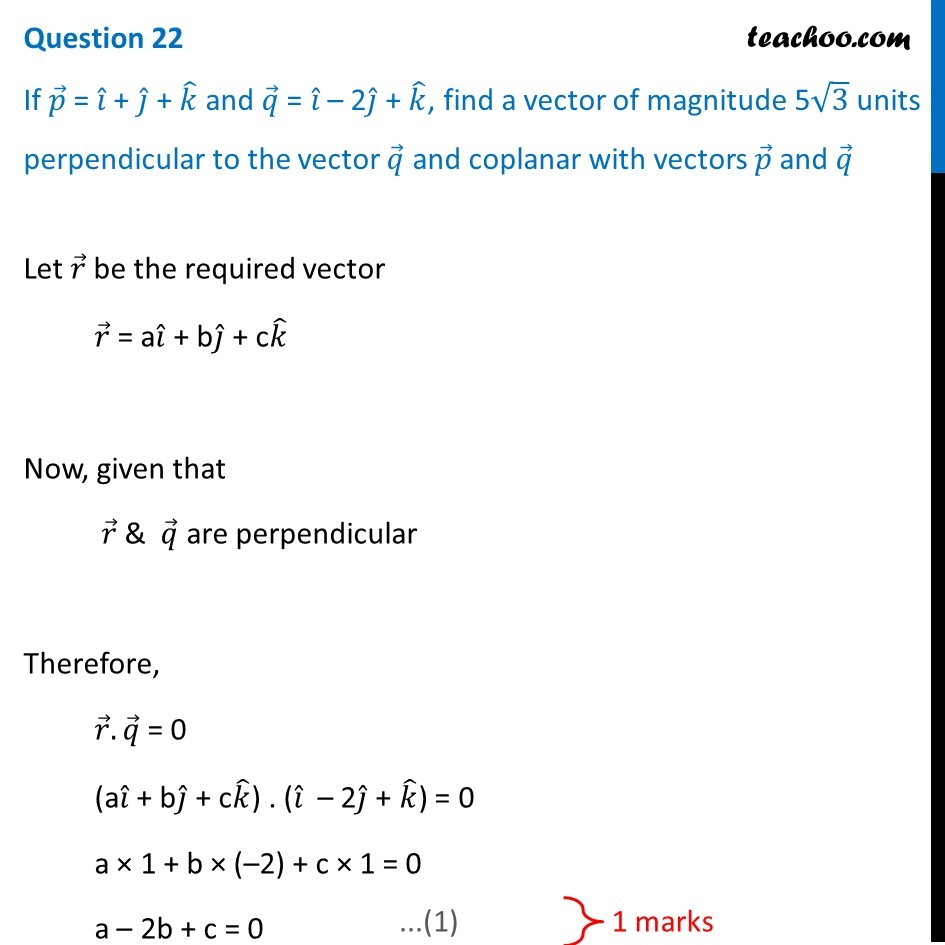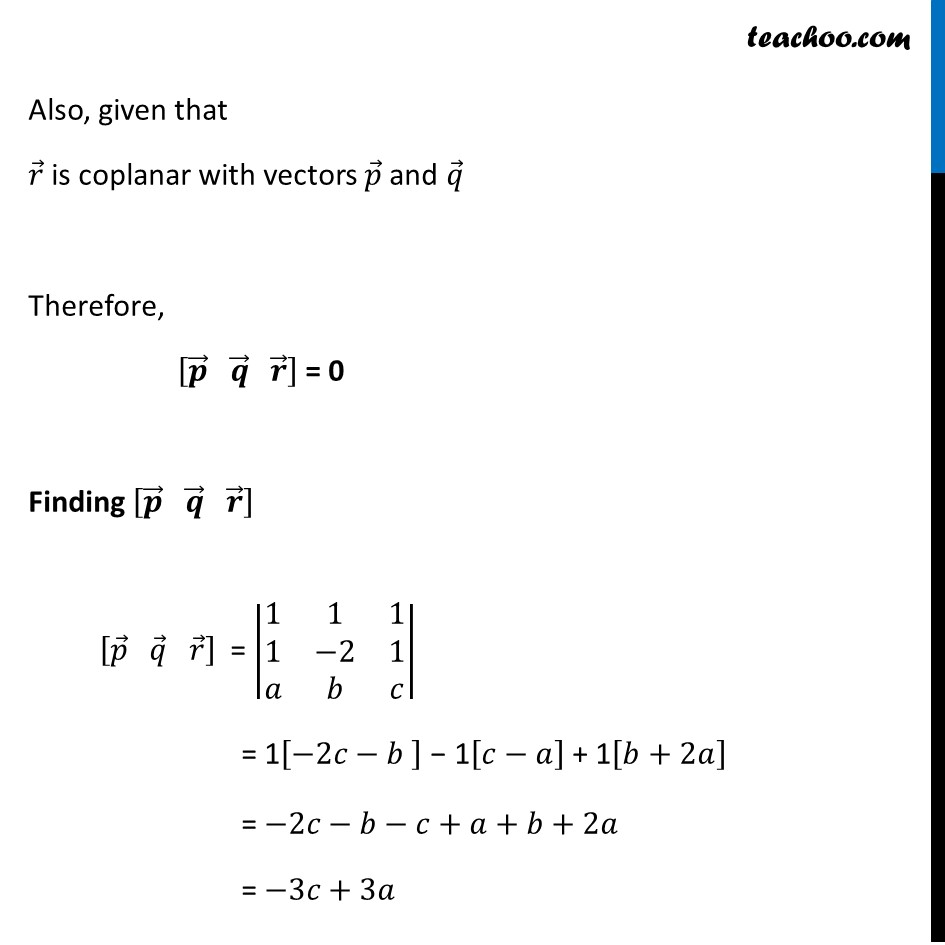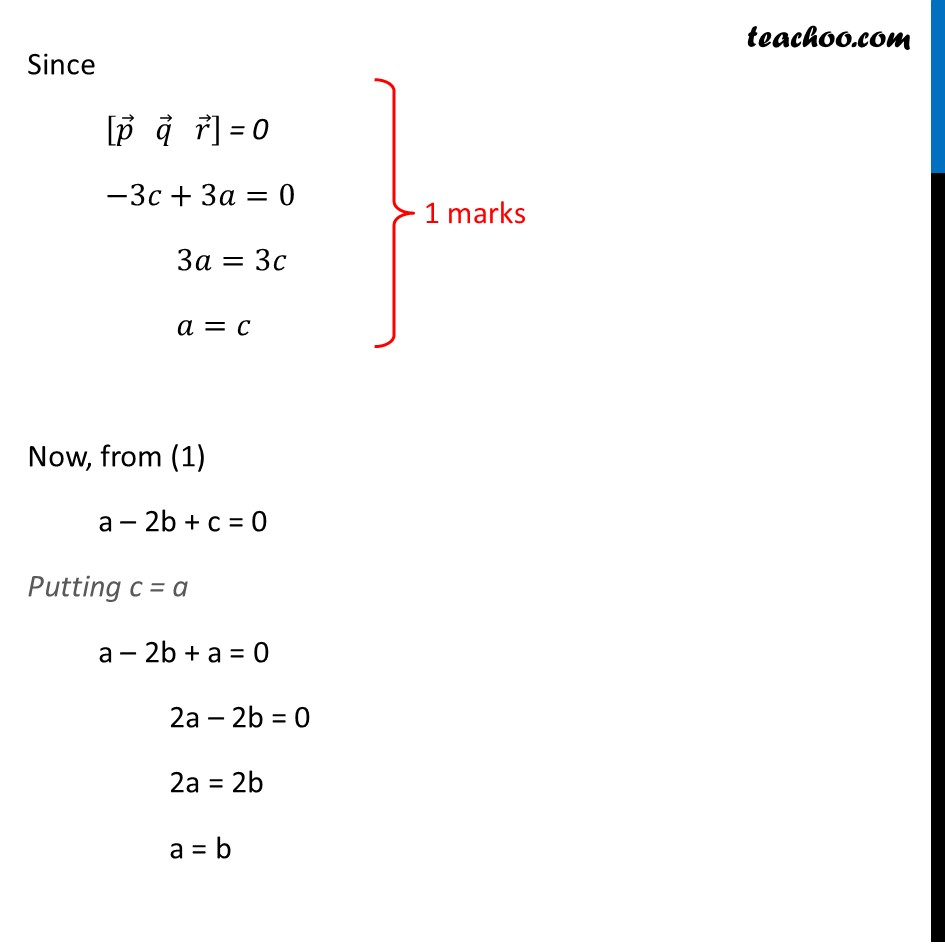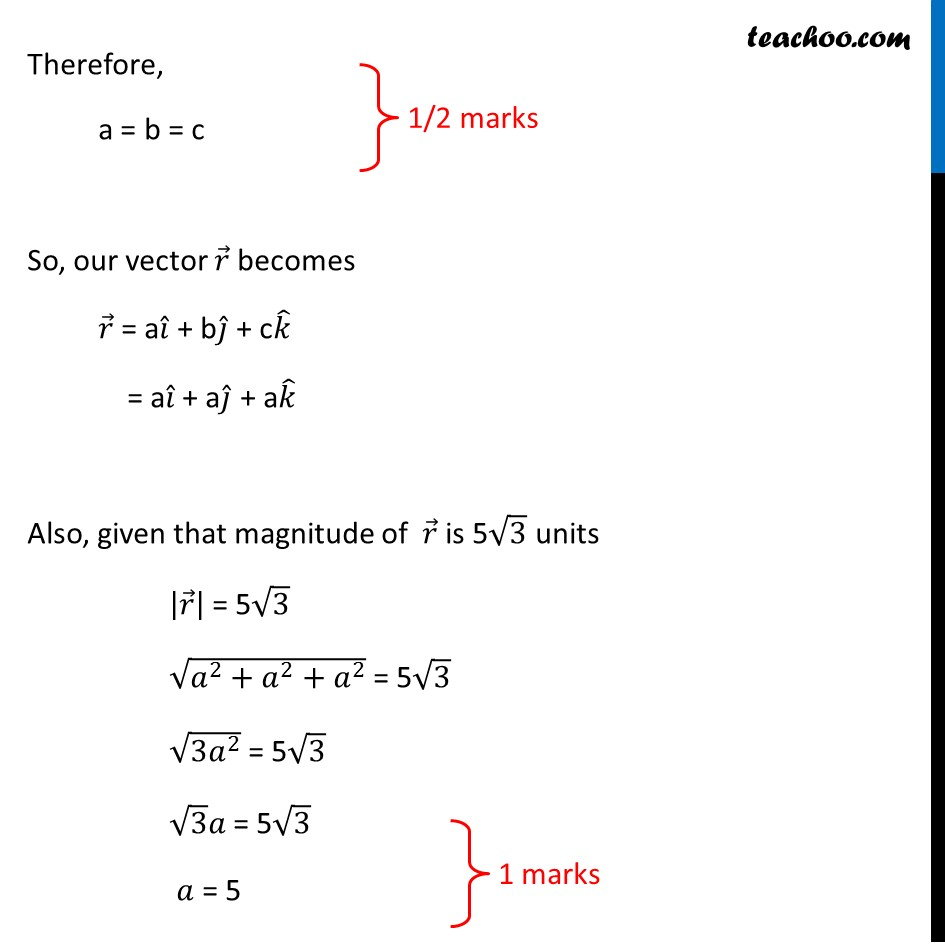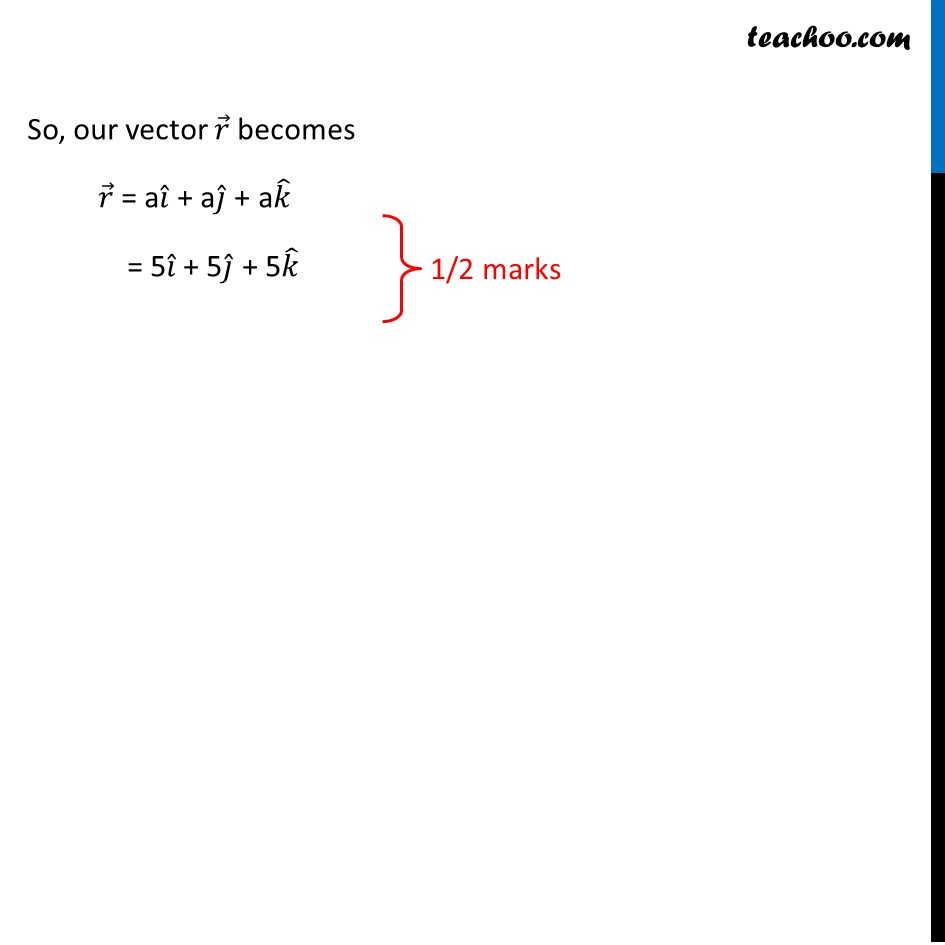Learn in your speed, with individual attention - Teachoo Maths 1-on-1 Class

### Transcript

Question 22 If 𝑝 ⃗ = 𝑖 ̂ + 𝑗 ̂ + 𝑘 ̂ and 𝑞 ⃗ = 𝑖 ̂ – 2𝑗 ̂ + 𝑘 ̂, find a vector of magnitude 5√3 units perpendicular to the vector 𝑞 ⃗ and coplanar with vectors 𝑝 ⃗ and 𝑞 ⃗ Let 𝑟 ⃗ be the required vector 𝑟 ⃗ = a𝑖 ̂ + b𝑗 ̂ + c𝑘 ̂ Now, given that 𝑟 ⃗ & 𝑞 ⃗ are perpendicular Therefore, 𝑟 ⃗.𝑞 ⃗ = 0 (a𝑖 ̂ + b𝑗 ̂ + c𝑘 ̂) . (𝑖 ̂ – 2𝑗 ̂ + 𝑘 ̂) = 0 a × 1 + b × (–2) + c × 1 = 0 a – 2b + c = 0 Also, given that 𝑟 ⃗ is coplanar with vectors 𝑝 ⃗ and 𝑞 ⃗ Therefore, [𝒑 ⃗" " 𝒒 ⃗" " 𝒓 ⃗ ] = 0 Finding [𝒑 ⃗" " 𝒒 ⃗" " 𝒓 ⃗ ] [𝑝 ⃗" " 𝑞 ⃗" " 𝑟 ⃗ ] = |■8(1&1&1@1&−2&1@𝑎&𝑏&𝑐)| = 1[−2𝑐−𝑏 ] − 1[𝑐−𝑎] + 1[𝑏+2𝑎] = −2𝑐−𝑏−𝑐+𝑎+𝑏+2𝑎 = −3𝑐+3𝑎 Since [𝑝 ⃗" " 𝑞 ⃗" " 𝑟 ⃗ ] = 0 −3𝑐+3𝑎=0 3𝑎=3𝑐 𝑎=𝑐 Now, from (1) a – 2b + c = 0 Putting c = a a – 2b + a = 0 2a – 2b = 0 2a = 2b a = b Therefore, a = b = c So, our vector 𝑟 ⃗ becomes 𝑟 ⃗ = a𝑖 ̂ + b𝑗 ̂ + c𝑘 ̂ = a𝑖 ̂ + a𝑗 ̂ + a𝑘 ̂ Also, given that magnitude of 𝑟 ⃗ is 5√3 units |𝑟 ⃗ | = 5√3 √(𝑎^2+𝑎^2+𝑎^2 ) = 5√3 √(〖3𝑎〗^2 ) = 5√3 √3 𝑎 = 5√3 𝑎 = 5 So, our vector 𝑟 ⃗ becomes 𝑟 ⃗ = a𝑖 ̂ + a𝑗 ̂ + a𝑘 ̂ = 5𝑖 ̂ + 5𝑗 ̂ + 5𝑘 ̂